# Logistic Equation

The logistics equation is a differential equation that models population growth. Often in practice a differential equation models some physical situtation, and you should read it'' as doing so.

Exponential growth: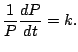This says that the relative (percentage) growth rate'' is constant. As we saw before, the solutions are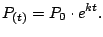Note that this model only works for a little while. In everyday life the growth couldn't actually continue at this rate indefinitely. This exponential growth model ignores limitations on resources, disease, etc. Perhaps there is a better model?

Over time we expect the growth rate should level off, i.e., decrease to 0. What about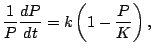(7.1)

where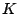is some large constant called the carrying capacity, which is much bigger than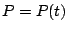at time 0. The carrying capacity is the maximum population that the environment can support. Note that if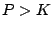, then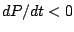so the population declines. The differential equation () is called the logistic model (or logistic differential equation). There are, of course, other models one could use, e.g., the Gompertz equation.

First question: are there any equilibrium solutions to (), i.e., solutions with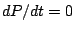, i.e., constant solutions? In order thatthen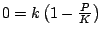, so the two equilibrium solutions are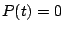and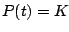.

The logistic differential equation () is separable, so you can separate the variables with one variable on one side of the equality and one on the other. This means we can easily solve the equation by integrating. We rewrite the equation as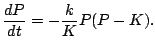Now separate: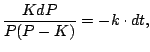and integrate both sides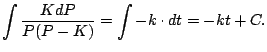On the left side we get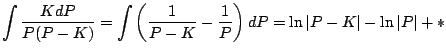Thus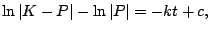so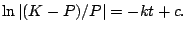Now exponentiate both sides: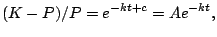where $A=e^c$Thus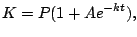so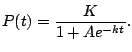Note that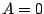also makes sense and gives an equilibrium solution. In general we have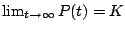. In any particular case we can determine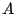as a function of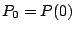by using that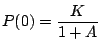so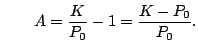William Stein 2006-03-15